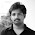I. 'BODMAS' Rule: This rule depicts the correct sequence in which the operations are to be executed, so as to find out the value of a given expression. Here, 'B' stands for 'Bracket', 'O' for 'of', 'D' for Division', 'M' for 'multiplication', 'A' for 'Addition' and 'S' for 'Subtraction'. Thus, in simplifying an expression, first of all the brackets must be removed, strictly in the order (),{} and []. After removing the brackets, we must use the following operations strictly in the order:
(i) of (ii) Division (iii) Multiplication (iv) Addition (v) Subtraction.
Eg: (-5)(4)(2)(-1/2)(3/4)=?
-5*4*2*-1/2*3/4= 15

II. Modulus of a Real Number: Modulus of a real number a is defined as
a|= a, if a>0 or
|a|= -a, if a<0
Thus, |5|= 5 and |-5|= -(-5)=5

III. Virnaculum (or Bar): When an expression contains Virnaculam, before applying the 'BODMAS' rule, we simplify the expression under the Virnaculum.

Exercise Questions
1.((469 + 174)2 - (469 - 174)2)/(469 x 174) = ?
a. 2
b. 4
c. 295
d. 643
Explanation: Given exp. = ((a + b)2 - (a - b)2)/ab
=4ab/ab = 4

2. David gets on the elevator at the 11th floor of a building and rides up at the rate of 57 floors per minute. At the same time, Albert gets on an elevator at the 51st floor of the same building and rides down at the rate of 63 floors per minute. If they continue travelling at these rates, then at which floor will their paths cross ?
a. 19
b. 28
c. 30
d. 37
Explanation: Suppose their paths cross after x minutes. Then, 11 + 57x = 51 - 63x => 120x = 40
x=1/3
Number of floors covered by David in (1/3) min. = (1/3)*57= 19
So, their paths cross at (11 +19) i.e., 30th floor.

3. A man has some hens and cows. If the number of heads be 48 and the number of feet equals 140, then the number of hens will be:
a. 22
b. 23
c. 24
d. 26
Explanation: Let the number of hens be x and the number of cows be y.
Then, x + y = 48 .... (i)
and 2x + 4y = 140 =>   x + 2y = 70 .... (ii)
Solving (i) and (ii) we get: x = 26, y = 22.
Therefore, The required answer = 26.

4. In a regular week, there are 5 working days and for each day, the working hours are 8. A man gets Rs. 2.40 per hour for regular work and Rs. 3.20 per hours for overtime. If he earns Rs. 432 in 4 weeks, then how many hours does he work for ?
a. 160
b. 175
c. 180
d. 195
Explanation: Suppose the man works overtime for x hours.
Now, working hours in 4 weeks = (5 * 8 * 4) = 160.
Therefore, 160 * 2.40 + x * 3.20 = 432
=> 3.20x = 432 - 384 = 48
=> x = 15.
Hence, total hours of work = (160 + 15) = 175.

5. One-third of Rahul's savings in National Savings Certificate is equal to one-half of his savings in Public Provident Fund. If he has Rs. 1,50,000 as total savings, how much has he saved in Public Provident Fund ?
a. Rs. 30,000
b.  Rs. 50,000
c. Rs. 60,000
d. Rs. 90,000
Explanation: Let savings in N.S.C and P.P.F. be Rs. x and Rs. (150000 - x) respectively. Then,
(1/3)x= (1/2)(150000-x)
x/3+x/2=75000
5x/6=75000
x=75000*6/5= 90000
Therefore, Savings in Public Provident Fund = Rs. (150000 - 90000) = Rs. 60000

6. A sum of Rs. 1360 has been divided among A, B and C such that A gets 2/3 of what B gets and B gets 1/4 of what C gets. B's share is:
a. Rs.120
b. Rs. 160
c. Rs.240
d. Rs.300
Explanation: Let C's share = Rs. x
Then, B's share = Rs. x/4; A's share = Rs.2/3 * x/4= Rs. x/6
Therefore x/6 + x/4 + x = 1360
17x/12 = 1360
x= 1360*12/17= Rs.960
Hence, B's share= Rs.960/4= Rs.240

7. If a-b= 3 and a2+b2=29, find the value of ab
a. 10
b. 12
c. 15
d. 18
Explanation: 2ab = (a2 + b2) - (a - b)2
= 29 - 9 = 20
=> ab = 10.

8. If 45-[28-{37-(15-*)}]= 58, then * is equal to:
a. -29
b. -19
c. 19
d. 29
1.